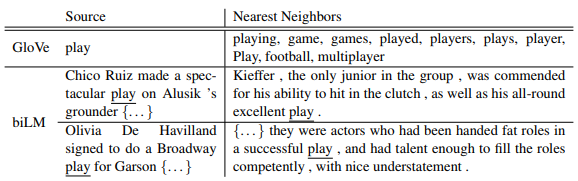# Deep Contextualized Word Representations

## WHY?

Former word representations such as Word2Vec or GloVe didn’t contained linguistic context.

## WHAT?

This paper suggests embedding from language model(ELMO) to include context information of word. Assume that x is context independent representation (token embedding or CNN over characters). Bi-directional l layers LSTM are used to predict previous or next token. Each hidden vector of LSTM can be viewed as a representation for a word (2L+1 representations for a word). These representations are weighted sum to be used as task specific representation. R_k = \{x_{k, h}, h^{\leftarrow}_{k,j}, h^{\rightarrow}_{k,j}|j = 1, ..., L\}\\ = \{h^{LM}_{k,j}|j = 0, ..., L\}\\ ELMo^{task}_k = E(R_k, \Theta^{task}) = \gamma^{task}\sum^L_{j=0}s^{task}_j h^{LM}_{k,j}$R_k = \{x_{k, h}, h^{\leftarrow}_{k,j}, h^{\rightarrow}_{k,j}|j = 1, ..., L\}\\ = \{h^{LM}_{k,j}|j = 0, ..., L\}\\ ELMo^{task}_k = E(R_k, \Theta^{task}) = \gamma^{task}\sum^L_{j=0}s^{task}_j h^{LM}_{k,j}$ Task specific parameters can be learned in task. Layer normalization can be used to each representation when weighting.
Using the pretrained biLM, we can make 2L+1 representations for each word in task corpus and weighted sum to get a fixed representation. This representation can be used either only in the input or both in the input and output.

## So?

ELBO achieved state-of-the-art result in nealy all possible NLP task including QA, textual entailment, semantic role labeling, coreference resolution, named entity extraction and sentimant analysis.Since word representation can be differ in context, ELMo have effect of word sense disambiguation and contain information of POS tagging. Also, training model using ELMo was much faster(98%) to achieve SOTA results.

## Critic

Another incredible breakthrough in NLP.

Powered by aiden